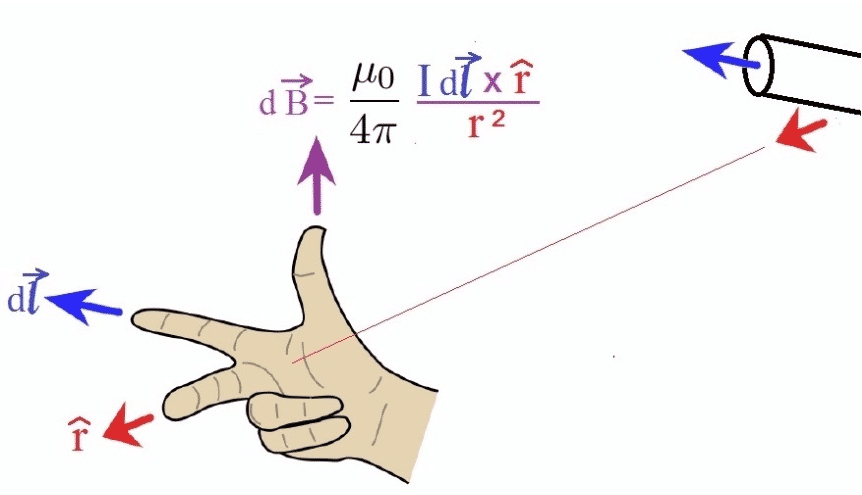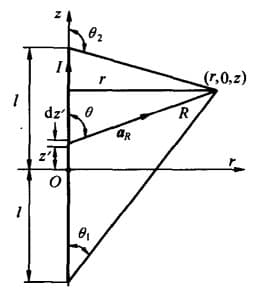# 磁感应强度

## 比奥-萨伐尔定律$$\bd{B}=\frac{\mu_0}{4\pi}\int_{c'} \frac{I\dif \bd{l}'\times \bd{a}_R}{R^2}\\$$ 根据几何关系，我们可以写出：
$$\begin{cases} \dif \bd{l}'=\dif \bd{z}'\\ z'=z-\frac{r}{\tan\theta}\\ \dif z'= r\csc^2 \theta\dif \theta\\ \dif \bd{z}'=\bd{a}_z r\csc^2 \theta\dif \theta\\ \bd{a}_R=\bd{a}_r \sin\theta+\bd{a}_z\cos\theta\\ \dif z'\times \bd{a}_R=\bd{a}_\varphi r \csc^2\theta\sin\theta \dif \theta\\ R=r\csc\theta \end{cases}$$ 将上面最后两项代入比奥-萨伐尔定律：
\begin{align} \bd{B}&=\frac{\mu_0}{4\pi}\int_{c'} \frac{I\dif \bd{l}'\times \bd{a}_R}{R^2}\\ &=\frac{\mu_0 I}{4\pi} \int_{\theta_1}^{\theta_2} \frac{r\csc^2\theta\sin\theta\dif \theta}{r^2\csc^2 \theta}\\ &=\frac{\mu_0 I}{4\pi r} \int_{\theta_1}^{\theta_2} \sin\theta\dif\theta\\ &=\frac{\mu_0 I}{4\pi r} (\cos\theta_1 - \cos \theta_2) \end{align} 由上可以推出无限长直导线的磁场强度为 $\bd{B}=\frac{\mu_0 I}{2\pi r} \bd{a}_\varphi$